# Test: Network Topology

## 10 Questions MCQ Test Topicwise Question Bank for Electronics Engineering | Test: Network Topology

Description
Attempt Test: Network Topology | 10 questions in 30 minutes | Mock test for Electrical Engineering (EE) preparation | Free important questions MCQ to study Topicwise Question Bank for Electronics Engineering for Electrical Engineering (EE) Exam | Download free PDF with solutions
QUESTION: 1

### A possible tree of the topology equivalent of the network shown below is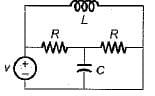Solution:

Given network has 4 number of nodes and 5 number of branches.
Hence, it’s tree will be as shown below.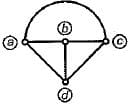QUESTION: 2

Solution:
QUESTION: 3

### The number of twigs and links in the graph obtained for the given network will be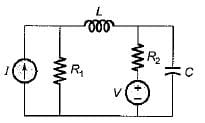Solution:

For the given network, number of nodes, n = 3 and number of branches = 4 = b.
Hence, twig s = n - 1 = 3 - 1 = 2 and links = b - { n - 1) = 4 - ( 3 - 1 ) = 2

QUESTION: 4

Consider the following statements concerned with the incidence matrix of a graph:
1. Determinant of the incident matrix of a closed loop is non-zero.
2. Algebraic sum of the row entires of an incidence matrix is zero.
3. If branch j in the graph is not incident at node aij = 0,

Which of the above statements is/are true?

Solution:
QUESTION: 5

The number of edges in a complete graph of n-vertices is

Solution:

Number of edge =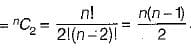QUESTION: 6

The number of-branches and nodes in the graph are respectively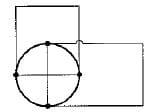Solution:

n - 5 and b = 10

QUESTION: 7

Assertion (A): When all the elements (i.e. resistors, inductors, capacitors etc.) in a network are replaced by lines with circles or dots at both ends, the configuration is then called the graph of the network.

Reason (R): The graph of any network is drawn by keeping all the points of intersection of two or more branches and representing the network elements of lines, voltages and current sources by their internal impedances.

Solution:
QUESTION: 8

The graph of a network has N number of nodes and B is the number of branches in it.
Match the List -I and List-II and select the correct answer using the codes given below the lists: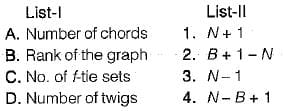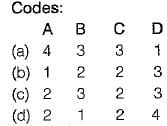Solution:
QUESTION: 9

Two graphs are said to be isomorphic graph if their incidence matrices

Solution:
QUESTION: 10

Assertion (A): The analysis of network is done using Kirchhoffs laws when the values of each element of the network remains constant for all the values of time.

Reason (R): The analysis of a network is done using graph theory when the values of each element of the network are changed with time.

Solution:

Assertion is true because if the values of each element is changed w.r.t. time, then every time a fresh KVL and KCL equations are to be written for analysis of the network.
Reason is also true but, the correct explanation of assertion is as explained above, Hence, Reason (R) is not the correct explanation of (A).Use Code STAYHOME200 and get INR 200 additional OFF Use Coupon Code